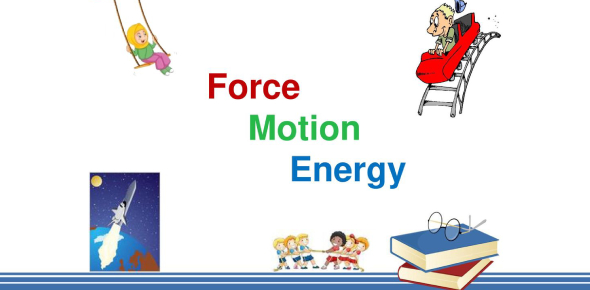# Physics Practice Questions From Forces, Energy And Motion! Quiz

10 Questions | Attempts: 481
ShareSettings.

• 1.
A wheeled cart is moving eastward at a speed of 1 meter per second when it is acted on by a 30-newton force to the north and a 30-newton force to the south. Which of the following BEST explains why the cart’s speed and direction stay the same?
• A.

The new forces are perpendicular to the main force.

• B.

The new forces are balanced.

• C.

The new forces are smaller than the main force.

• D.

The new forces are in opposite directions.

• 2.
Two dogs are pulling on opposite ends of a rope. The dog on the left is pulling with a force of 25 newtons. The dog on the right is pulling with a force of 60 newtons. What will be the resulting motion?
• A.

The dogs will move toward the right.

• B.

The dogs will move toward the left.

• C.

The dogs will not move.

• D.

Both dogs will move in the direction that they are pulling.

• 3.
A truck carrying a box is traveling 60 kilometers per hour. Suddenly, the truck brakes to a stop. Which choice best explains what will happen to the box?
• A.

The box will slide off the back because for every action there is an equal and opposite reaction.

• B.

The box will not move because objects at rest tend to stay at rest.

• C.

The box will keep moving forward because objects in motion tend to stay in motion.

• D.

The box will gain speed because, for objects of a given mass, greater force results in a greater acceleration.

• 4.
What part of Newton’s first law of motion is demonstrated when a magician pulls the tablecloth out from under a set of dishes?
• A.

An object in motion will tend to be acted upon by an outside force.

• B.

An object in motion tends to come to rest.

• C.

An object in motion will tend to stay in motion.

• D.

An object at rest will tend to stay at rest.

• 5.
Look at the illustrations. In the first, the truck is traveling 30 miles per hour. In the second, the truck has braked to a stop. Which of Newton’s laws of motion explains what happens next?
• A.

For every action there is an equal and opposite reaction.

• B.

Objects at rest tend to stay at rest.

• C.

Objects in motion tend to stay in motion.

• D.

For objects of a given mass, greater force results in a greater acceleration.

• 6.
The man who introduced the idea that all of the continents of the Earth were at one timejoined to make one large land mass was -
• A.

Arthur Holmes

• B.

Harry Hess

• C.

John Dalton

• D.

Alfred Wegener

• 7.
The most recently formed crust of the Earth would be found -
• A.

Where plates moving sideways passed each other

• B.

Where one plate is going under another plate

• C.

Where two plates are pushing directly toward each other

• D.

Where two plates are moving away from each other in opposite directions

• 8.
Which of these landforms would most likely be found at a divergent boundary?
• A.

Volcanic island

• B.

Mid-ocean ridge

• C.

Fault line

• D.

Mountain

• 9.
Which of these landforms is most likely to be found at a convergent boundary?
• A.

Fault line

• B.

Mountain

• C.

Mid-ocean ridge

• D.

Rift valley

• 10.
Martin gathered a toy car, a ramp, a stopwatch, a meter stick, and a variety of weights. What is he likely testing?
• A.

How the angle of a ramp affects the speed of the car

• B.

How friction affects the speed of the car

• C.

How equal forces work on the placement of the car and the ramp

• D.

How mass affects the speed of the car

## Related TopicsBack to top
×

Wait!
Here's an interesting quiz for you.